##### The Japanese Bonsai specialist
Direct order Contact Help / Services Newsletter# Tool kit n°1

› Bonsai tools › Bonsai toolkits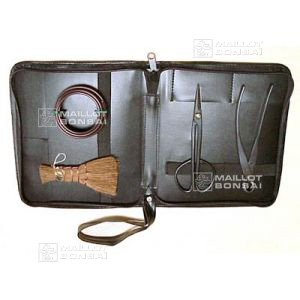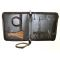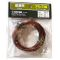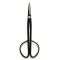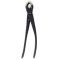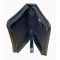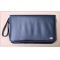ref. : 5453

100,00

Available quantity : 5Order

###### Description

1 kit in black skai 235*205 mm. This is a brilliant starter kit for bonsaists and a good gift idea. The smart faux leather zipped case comes with top quality tools, including a coconut palm brush, binding wire 80 gr, 1 pair of straight scissors 180 mm and 1 digging tool 180 mm. The case can accommodate 7 tools in its elasticated compartments and the wrist strap makes it easy to carry.

#tool 3.4 #toolkits 2.8 #bonsai 2.6 #tools 2.5 #case 2.4 #compartments 2.2 #accommodate 2.1 #elasticated 2.1 #including 1.9 #bonsaists 1.9

Formule
(( ROUND((CHAR_LENGTH(b.article_nom)-CHAR_LENGTH(REPLACE(b.article_nom, 'tool', '')))/LENGTH('tool')) + ROUND((CHAR_LENGTH(b.article_description)-CHAR_LENGTH(REPLACE(b.article_description, 'tool', '')))/LENGTH('tool')) ) * 3.4) + (( ROUND((CHAR_LENGTH(b.article_nom)-CHAR_LENGTH(REPLACE(b.article_nom, 'tools', '')))/LENGTH('tools')) + ROUND((CHAR_LENGTH(b.article_description)-CHAR_LENGTH(REPLACE(b.article_description, 'tools', '')))/LENGTH('tools')) ) * 2.5) + (( ROUND((CHAR_LENGTH(b.article_nom)-CHAR_LENGTH(REPLACE(b.article_nom, 'case', '')))/LENGTH('case')) + ROUND((CHAR_LENGTH(b.article_description)-CHAR_LENGTH(REPLACE(b.article_description, 'case', '')))/LENGTH('case')) ) * 2.4) + (( ROUND((CHAR_LENGTH(b.article_nom)-CHAR_LENGTH(REPLACE(b.article_nom, 'compartments', '')))/LENGTH('compartments')) + ROUND((CHAR_LENGTH(b.article_description)-CHAR_LENGTH(REPLACE(b.article_description, 'compartments', '')))/LENGTH('compartments')) ) * 2.2) + (( ROUND((CHAR_LENGTH(b.article_nom)-CHAR_LENGTH(REPLACE(b.article_nom, 'accommodate', '')))/LENGTH('accommodate')) + ROUND((CHAR_LENGTH(b.article_description)-CHAR_LENGTH(REPLACE(b.article_description, 'accommodate', '')))/LENGTH('accommodate')) ) * 2.1) + (( ROUND((CHAR_LENGTH(b.article_nom)-CHAR_LENGTH(REPLACE(b.article_nom, 'elasticated', '')))/LENGTH('elasticated')) + ROUND((CHAR_LENGTH(b.article_description)-CHAR_LENGTH(REPLACE(b.article_description, 'elasticated', '')))/LENGTH('elasticated')) ) * 2.1) + (( ROUND((CHAR_LENGTH(b.article_nom)-CHAR_LENGTH(REPLACE(b.article_nom, 'including', '')))/LENGTH('including')) + ROUND((CHAR_LENGTH(b.article_description)-CHAR_LENGTH(REPLACE(b.article_description, 'including', '')))/LENGTH('including')) ) * 1.9) + (( ROUND((CHAR_LENGTH(b.article_nom)-CHAR_LENGTH(REPLACE(b.article_nom, 'brilliant', '')))/LENGTH('brilliant')) + ROUND((CHAR_LENGTH(b.article_description)-CHAR_LENGTH(REPLACE(b.article_description, 'brilliant', '')))/LENGTH('brilliant')) ) * 1.9) + (( ROUND((CHAR_LENGTH(b.article_nom)-CHAR_LENGTH(REPLACE(b.article_nom, 'bonsaists', '')))/LENGTH('bonsaists')) + ROUND((CHAR_LENGTH(b.article_description)-CHAR_LENGTH(REPLACE(b.article_description, 'bonsaists', '')))/LENGTH('bonsaists')) ) * 1.9) + (( ROUND((CHAR_LENGTH(b.article_nom)-CHAR_LENGTH(REPLACE(b.article_nom, 'straight', '')))/LENGTH('straight')) + ROUND((CHAR_LENGTH(b.article_description)-CHAR_LENGTH(REPLACE(b.article_description, 'straight', '')))/LENGTH('straight')) ) * 1.8)

## Secure payment## Delivery

Our logistic partners :04 74 55 23 48
Pépinière MAILLOT-BONSAÏ
Le Bois Frazy
01990 RELEVANT - FRANCE
on appointment